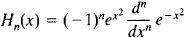# Hermite Polynomials

(redirected from Hermitian polynomials)

## Hermite polynomials

[er′mēt ‚päl·ə′nō·mē·əlz]
(mathematics)
A family of orthogonal polynomials which arise as solutions to Hermite's differential equation, a particular case of the hypergeometric differential equation.

## Hermite Polynomials

a special system of polynomials of successively increasing degree. For n = 0,1, 2,..., the Hermite polynomials Hn (x) are defined by the formulaIn particular, H0 = 1, H1 = 2x, H2 = 4x2 – 2, H3 = 8x3 – 12x, and H4 = 16x4 – 48x2 + 12.

Hermite polynomials are orthogonal on the entire x-axis with respect to the weight function

e –x2

(seeORTHOGONAL POLYNOMIAL). They satisfy the differential equation

y″ – 2xy′ + 2ny = 0

and the recursion formulas

Hn+1 (x ) – 2xHn (x ) + 2nHn-1 (x ) = 0

H′n(x ) – 2nH–1(x ) = 0

Also sometimes called Hermite polynomials are polynomials that differ from those given above by certain factors dependent on n; sometimes

e–x2/2

is used as the weight function. The basic properties of the system were studied by P. L. Chebyshev in 1859 and C. Hermite in 1864.

References in periodicals archive ?
The convergence of the DFE element was compared against the 12-DOF plate finite element, which utilizes Hermitian polynomials. For this study, the mesh density was increased from a single element to 4 elements (2 by 2), and to 25 elements (5 by 5).

Site: Follow: Share:
Open / Close